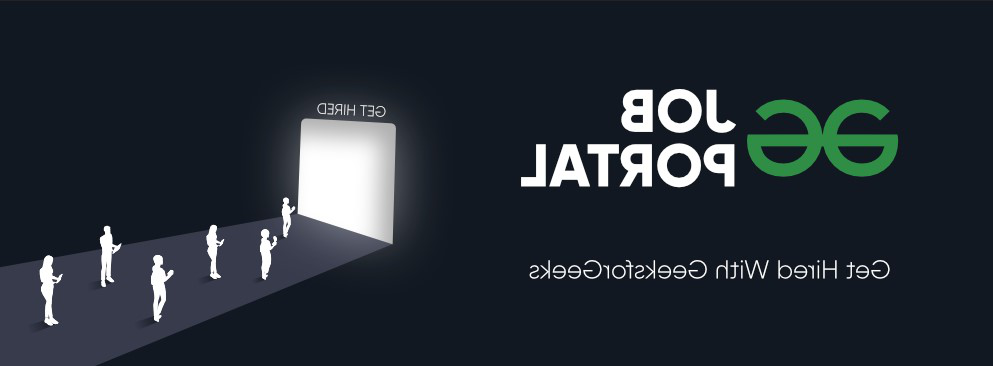# Python PyTorch – RandomHorizontalFlip() Function

• Last Updated : 10 Jun, 2022

In this article, we will discuss the RandomHorizontalFlip() Method in PyTorch Python.

## RandomHorizontalFlip() method

RandomHorizontalFlip() method of torchvision.transforms module is used to horizontally flip the given image at a random angle with a given probability. This method accepts a PIL and tensor image as input. The tensor image is a PyTorch tensor with shape [C, H, W], where C represents the number of channels and  H, W represents the height and width respectively. This method returns a horizontally flipped image and an original image if the probability P is 1 or 0 respectively, if P is in the range between 0 to 1 then P is the probability to return the horizontally flipped image.

Syntax: torchvision.transforms.RandomHorizontalFlip(p)(img)

Parameter:

• p: p is the probability of the image being flipped at a random angle.
• img: input image to be flipped.

Returns: This method returns a randomly flipped image  at a random angle.

The below image is used for demonstration:### Example 1:

In this example, we flip an image using RandomHorizontalFlip() Method when the probability is 1.

## Python3

 `# import required libraries``import` `torch``import` `torchvision.transforms as T``from` `PIL ``import` `Image`` ` `# read input image from computer``img ``=` `Image.``open``(``'a.png'``)`` ` `# define a transform``transform ``=` `T.RandomHorizontalFlip(p``=``1``)`` ` `# apply above defined transform to ``# input image``img ``=` `transform(img)`` ` `# display result``img.show()`

Output:### Example 2:

In this example, we flip an image using RandomHorizontalFlip() Method when the probability is in the range of 0 to 1.

## Python3

 `# import required libraries``import` `torch``import` `torchvision.transforms as T``from` `PIL ``import` `Image`` ` `# read input image from computer``img ``=` `Image.``open``(``'img.png'``)`` ` `# define a transform``transform ``=` `T.RandomHorizontalFlip(p``=``0.5``)`` ` `# apply above defined transform to ``# input image``img ``=` `transform(img)`` ` `# display result``img.show()`

Output:My Personal Notes arrow_drop_up